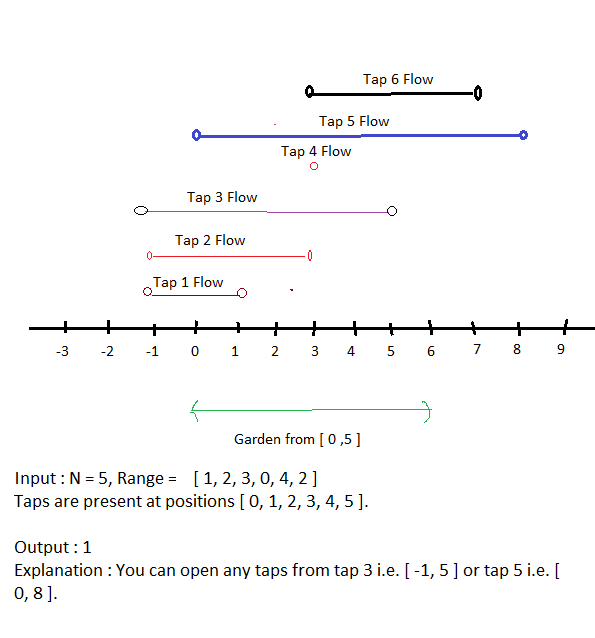New update is available. Click here to update.
Last Updated: 12 Nov, 2020
##### Minimum Number Of Taps To Water Garden
Hard
Problem statement#### You are given an integer N, and an array named “ranges” of size N + 1(0-indexed). The ith tap, if opened, can water the gardener from point (i - ranges[i]) to (i + ranges[i]) including both. The task is to find the minimum number of taps that should be open to water the whole garden, return -1 if the garden can not be watered.

##### Example :``````Can you solve the problem in O(N) time?
``````
##### Input Format:
``````The first line contains a single integer T representing the number of test cases.

The first line of each test case will contain the integer N.

The second and the last line of each test case will contain N single space-separated integers representing the elements of the array “ranges”.
``````
##### Output format :
``````For each test case, print a single integer representing the value of the minimum number of taps needed to open by the gardener to fill the whole garden.
``````
##### Note:
``````You do not need to print anything, it has already been taken care of. Just implement the given function.
``````
##### Constraints:
``````1 <= T <= 10
1 <= N <= 10^4
0 <= ranges[i] <= 100

Time Limit: 1 sec
``````Approaches

## 01Approach• The idea to approach the problem is to convert the Ranges array into a list of intervals and sort them.
• As we need to choose minimum taps so at first we will find the farthest tap which we will choose so that it will cover the 0th coordinate and after choosing this coordinate we will update the leftmost coordinate with the right of the current chosen tap. This will act as the left boundary for the remaining taps to cover. Also increment the tap count to be returned.
• Now we repeat the above 2nd step until we cover all the possible coordinates(when we reach a tap which will cover a range more than equal to the last coordinate of the garden) or if there is no possible tap left that can cover the leftmost updated coordinate.